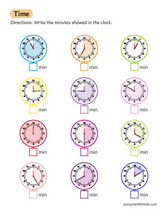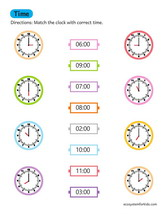# 2nd grade telling time math worksheets pdf

2nd grade telling time math worksheets pdf downloads. Learn to tell the time on clocks to the hour, minutes past, half past and a quarter past. Start by practicing these skills with the worksheets provided here. When this skill is mastered, try doing more complex tasks involving work problems. Have fun learning how to tell the time.

### TimeTime reading A.M. and P.M. worksheet

### Telling timeTell the time worksheet pdf

### Numbers

Counting and matching numbers

In this 2nd grade math lesson, children learn to relate the numbers on the clock face to intervals of five. They use this understanding to tell time to the nearest five minutes. Children learn about the terms before and after the clock and use subtraction to solve elapsed time problems. They also practice telling time with an analog clock.

## Telling time to the hour

One of the math skills your 2nd grade students will learn is how to tell time to the half hour and the hour. Fortunately, there are many resources to help you teach this important skill in second grade. Below you will find several worksheets to help your child become familiar with counting to the hour and half hour. Each worksheet includes a series of tasks for calculating the time and drawing the hands on a clock face.

## Telling time to the half hour

One of the first things students learn in 1st grade is how to tell time to the half hour. They will need to know how to set a clock to the hour, how to tell time in terms of half-hours, and how to read the hour hand. At the half hour, the hour hand is halfway between the minutes and hours, so it's crucial that they learn how to tell time with it.

## Telling time on an analog clock

First graders learn the basics of an analogue clock. They understand the concepts of hour, half hour, and hour interval and learn how to read both hands of a clock. Students learn to compare the hour and minute hands of two different clocks and relate them to each other. They can also relate analogue and digital clocks to each other. Using some of our resources, students can learn how to tell time on both analogue and digital clocks.

## Telling time on a digital clock

Although telling the time with a digital clock is not a Common Core standard, it is an important standard that all 2nd grade students learn. The goal is for second graders to understand how a clock works and be able to tell time to the hour and half hour. Digital clocks are pretty easy to read. What you see is actually the time.

Understanding the half hour

Children should be able to recognize the two half circles on a clock and relate them to the half hour. They should also know that the half hour is equal to 30 minutes and that a quarter hour is 15 minutes past the hour. A great tool for teaching first grade students about the half hour is this interactive math lesson found here. This lesson covers the terms hour, half hour, minute, and part of an hour. This concept is quite simple to understand.

## Using units of time vocabulary

Children are not yet able to understand the concept of minute, hour and half hour. They learn to tell time through experience. As parents and teachers, you should be mindful of using time vocabulary in your language use to help students understand the concept of time. The three expressions you should pay attention to first are hours, minutes, and seconds. Then also learn the terms half hour, quarter past, and finally morning and afternoon.